#Function Repository Resource:

# CombinatorEvolutionPlot

Visualize the evolution of a combinator expression

Contributed by: Wolfram Research
 ResourceFunction["CombinatorEvolutionPlot"][evol, type] renders the combinator evolution evol according to the specified type of visualization.

## Details and Options

The combinator evolution evol is a list of combinator expressions generated by application of rules in a defined traversal scheme on an initial combinator condition. Use either the resource function CombinatorEvolveList or the resource function CombinatorFixedPointList to generate possible combinator evolutions.
ResourceFunction["CombinatorEvolutionPlot"] accepts following type specifications:
 "StatesDisplay" displays the combinator evolution in a list, or multiple combinator evolutions in a list of lists, in a stylized way "ExpressionDepthPlot" displays an array plot showing the depths of subexpressions throughout the combinator evolution "SizeAndMatches" visualizes the leaf count of expressions and which leaves are changed by which rule at each step in evolution "UpdateDepthPlot" visualizes the depth at which rule applications occur relative to the overall leaf counts at each step in evolution "DepthAndUpdatePlot" visualizes depths of subexpressions throughout the combinator evolution, as well as which leaves are changed by which rule in evolution "DepthCuboidPlot" visualizes depths of subexpressions throughout the combinator evolution in 3D, as well as which leaves are changed by which rule in evolution "ArrayPlot" displays an array plot of evol "ArrayPlotLeftAssociative" displays an array plot wherein the application operator is left associative "ArrayPlotRightAssociative" displays an array plot wherein the application operator is right associative "ArrayPlotBracketsOnly" displays an array plot wherein only the brackets are shown "ArrayPlotPolishNotation" displays an array plot wherein each step is written in Polish notation "ArrayPlotReversePolishNotation" displays an array plot wherein each step is written in reverse Polish notation
ResourceFunction["CombinatorEvolutionPlot"] accepts options from the resource function CombinatorPlot (including options from ArrayPlot), Grid, ListPlot3D, ListStepPlot and Graphics3D, as well as the following:
 "SpanThickness" 0.2 the thickness of match indicator line in "SizeAndMatches", "DepthAndUpdatePlot" "IncludeDepthAxis" whether to show expression depth in 3D in "ExpressionDepthPlot" "DisplayStyles" styling for rendering evol in a grid in "StatesDisplay" "IncludeUpdateHighlighting" whether updates are indicated in "DepthCuboidPlot" "UpdateHighlightingStyles" styling for update highlighting

## Examples

### Basic Examples (12)

Display the fixed-point evolution of a combinator expression:

 In:=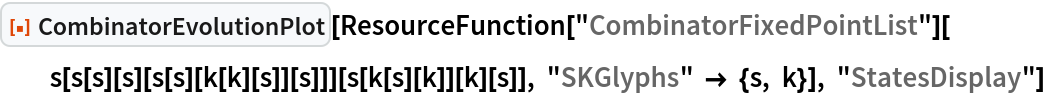Out=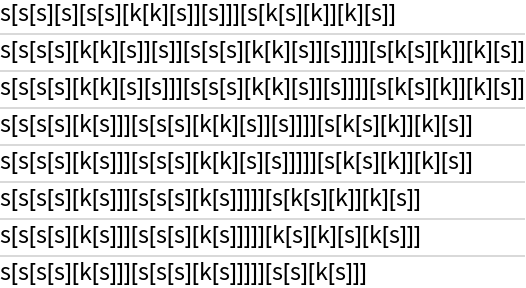Generate a plot showing the position depths in each expression of the combinator evolution:

 In:=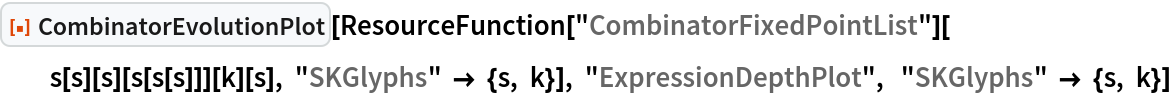Out=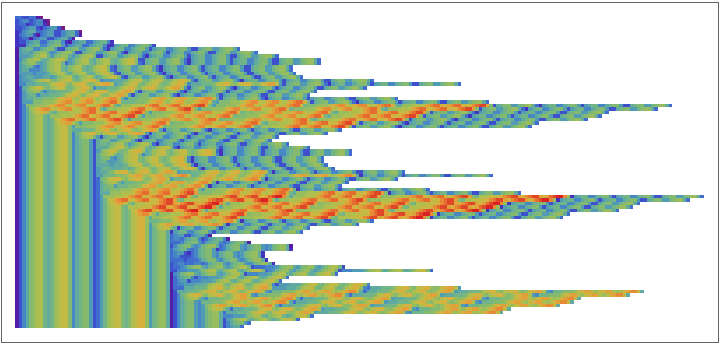Show the expression depths of the combinator evolution in 3D:

 In:=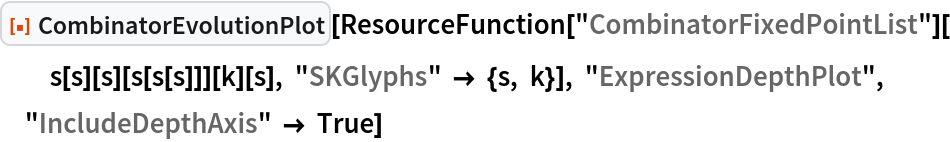Out=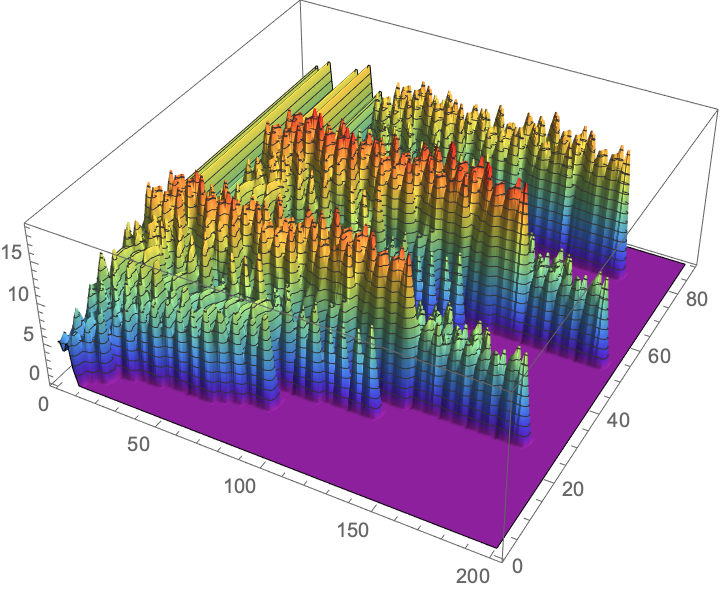Show the leaf count and position/type of the matches throughout the combinator evolution:

 In:=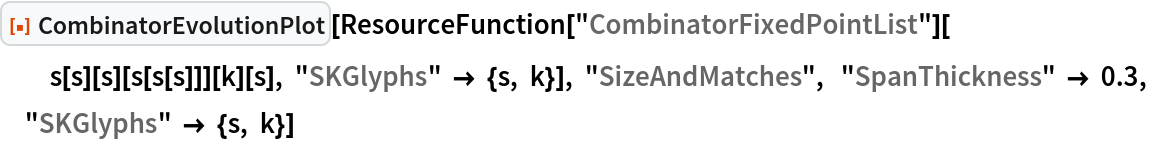Out=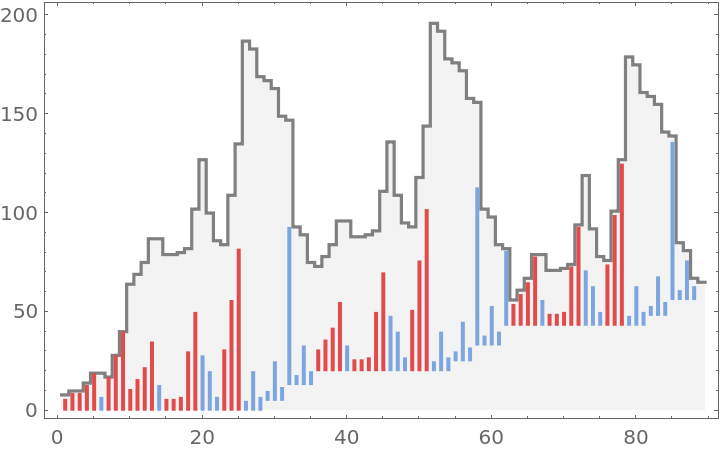Show the total depth of the combinator expressions and the depth at which the updates occur:

 In:=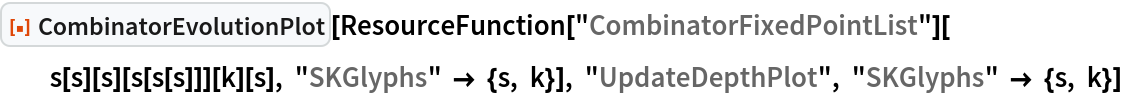Out=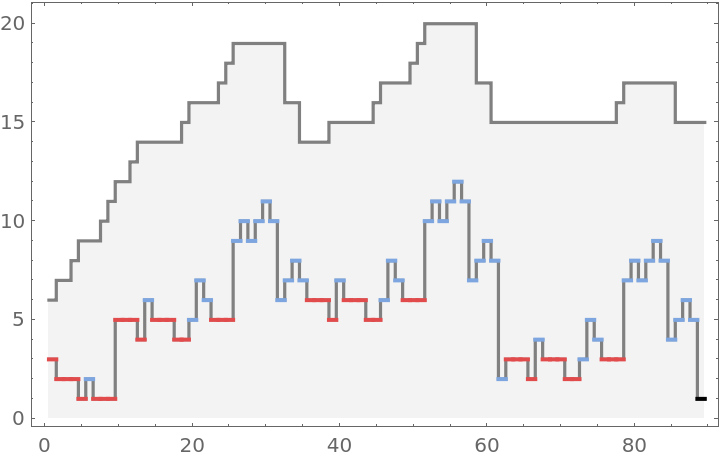Show the leaf count, depth of subexpressions, and position and type of the matches throughout the combinator evolution:

 In:=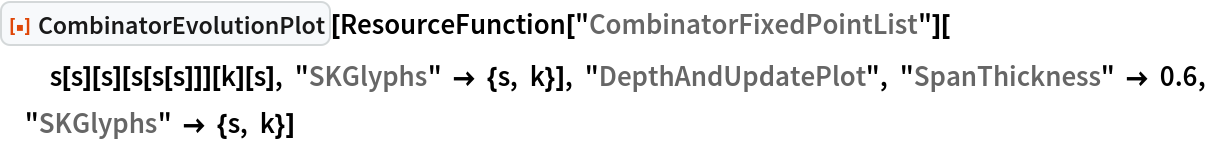Out=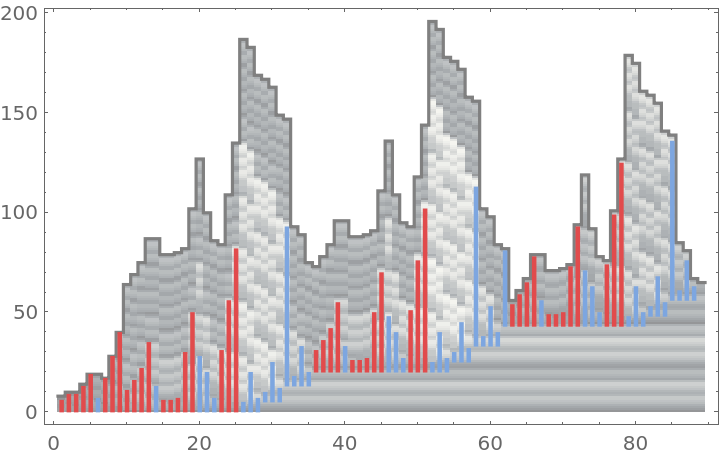Visualize the same information from the previous example in 3D:

 In:=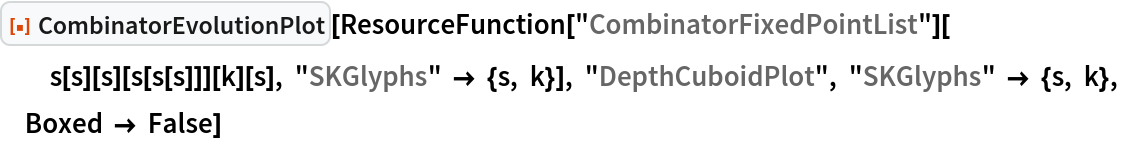Out=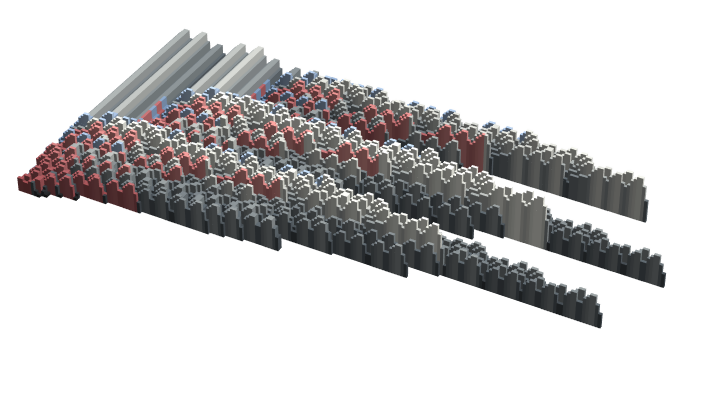Display an ArrayPlot of the combinator evolution in standard form:

 In:=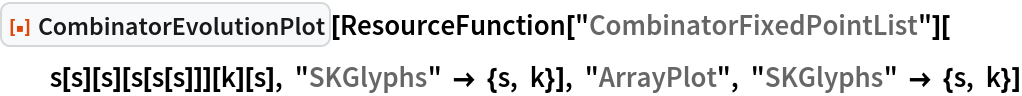Out=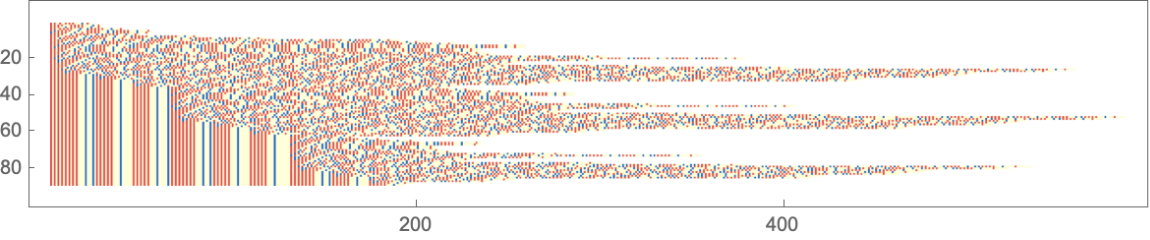Display an ArrayPlot of the combinator evolution with left associative combinator application:

 In:=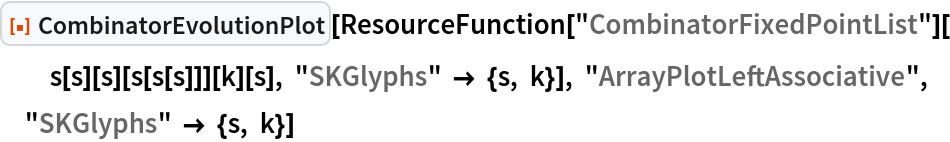Out=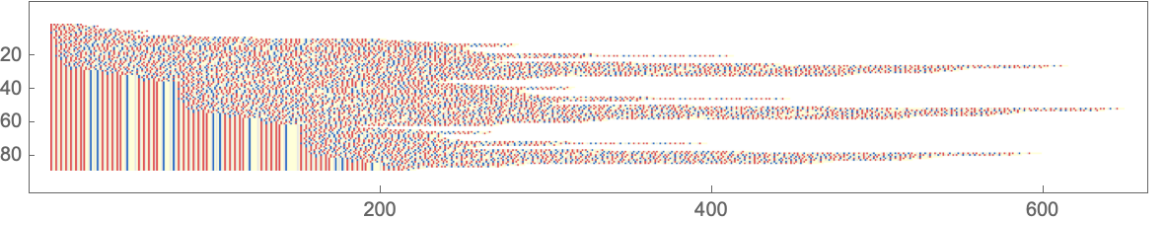Display an ArrayPlot of the combinator evolution with right associative combinator application:

 In:=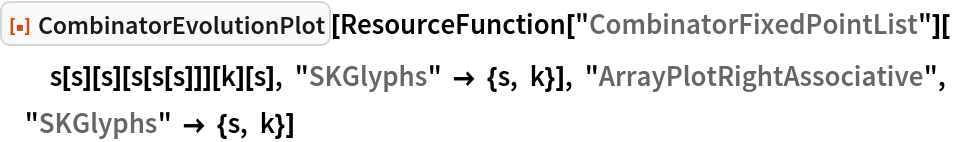Out=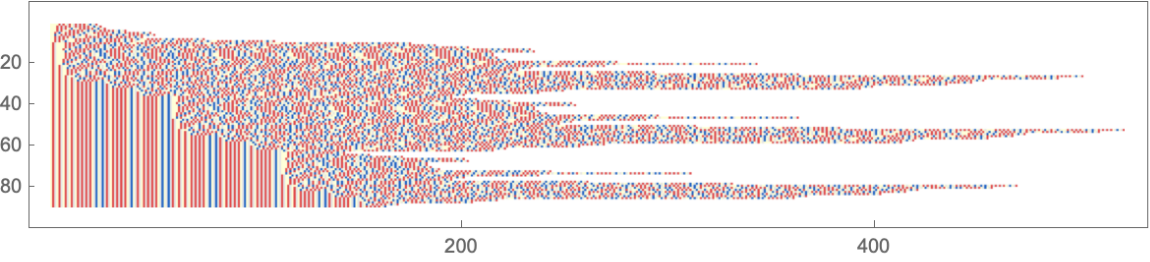Display an ArrayPlot of the combinator evolution with only the brackets kept in each expression:

 In:=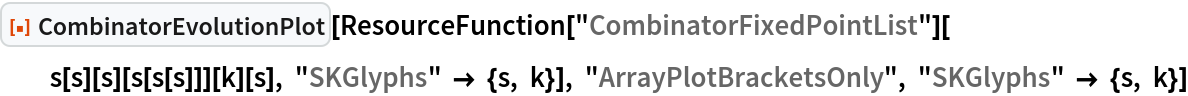Out=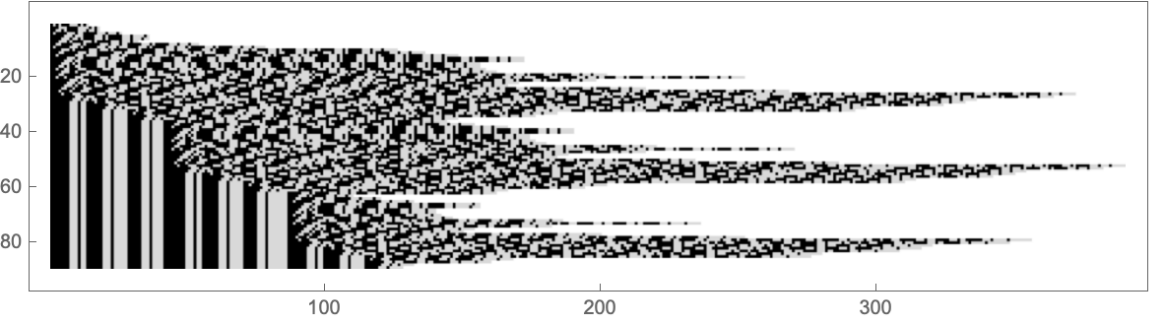Display an ArrayPlot of the combinator evolution in Polish notation:

 In:=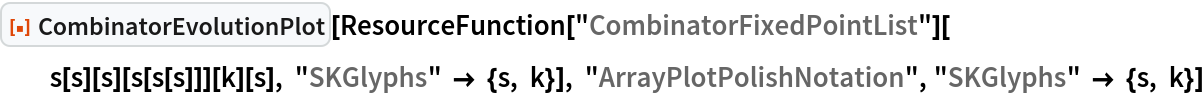Out=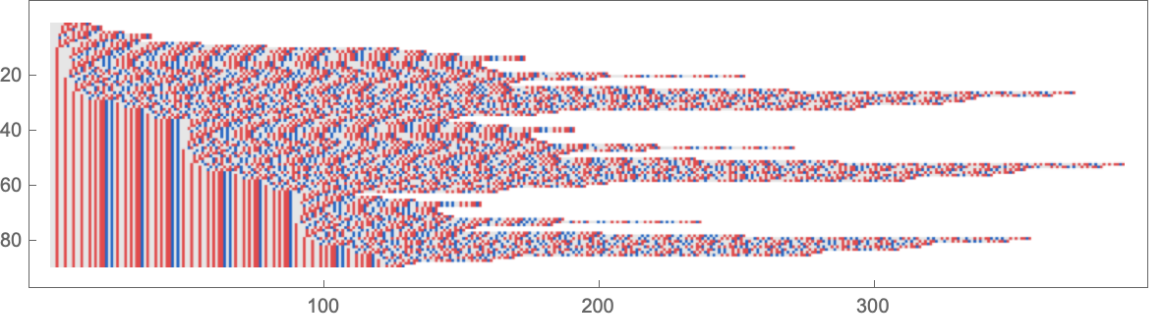Display an ArrayPlot of the combinator evolution in reverse Polish notation:

 In:=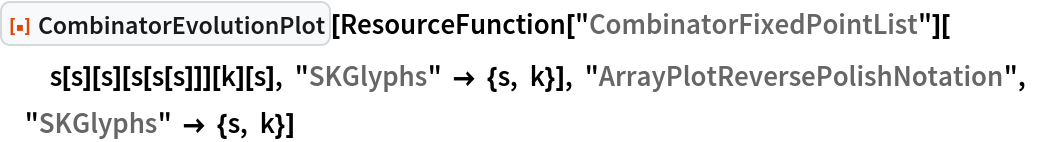Out=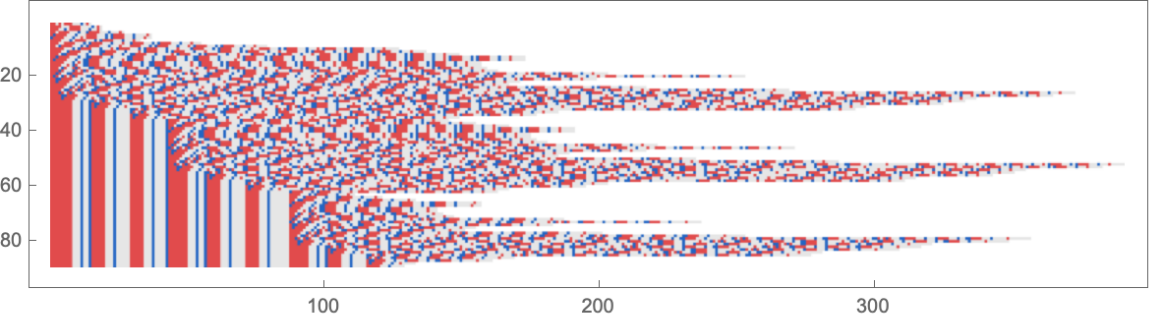### Scope (4)

Display a combinator evolution with left associative combinator application:

 In:=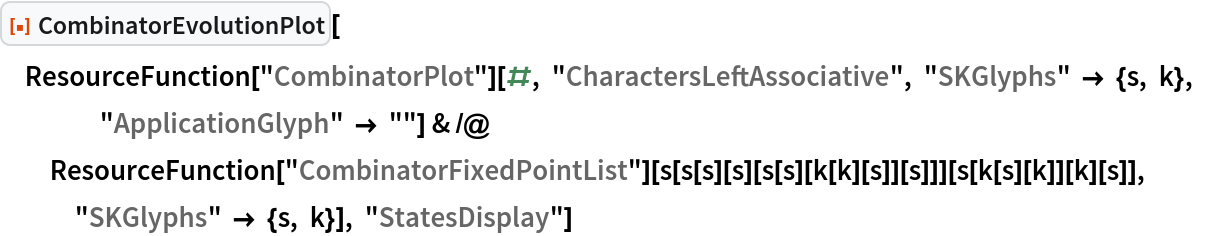Out=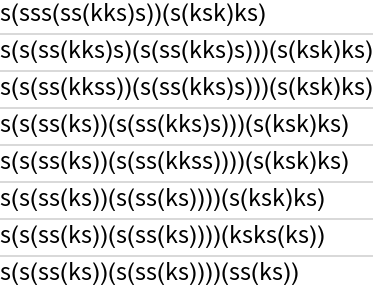Add styling to the same rendering of the combinator evolution:

 In:=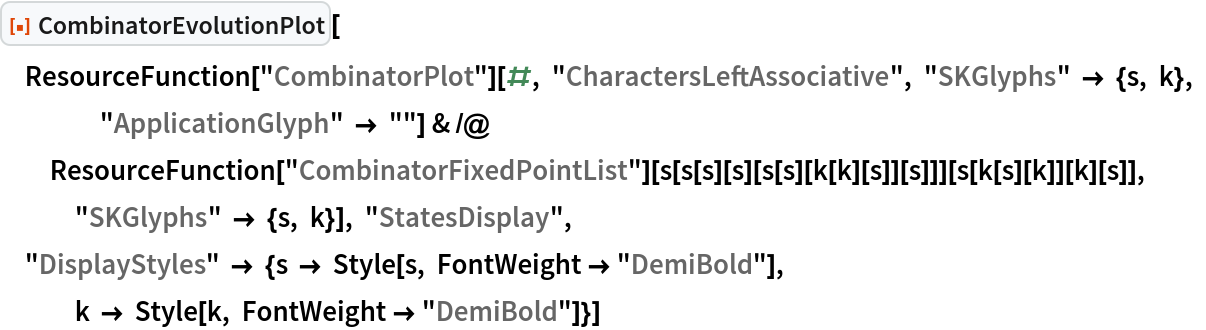Out=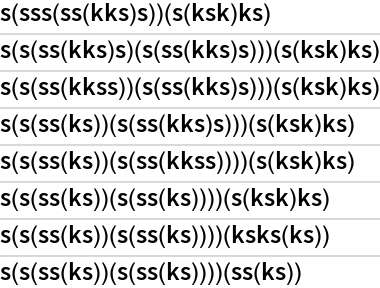Display a combinator expression evolved via two different evaluation schemes:

 In:=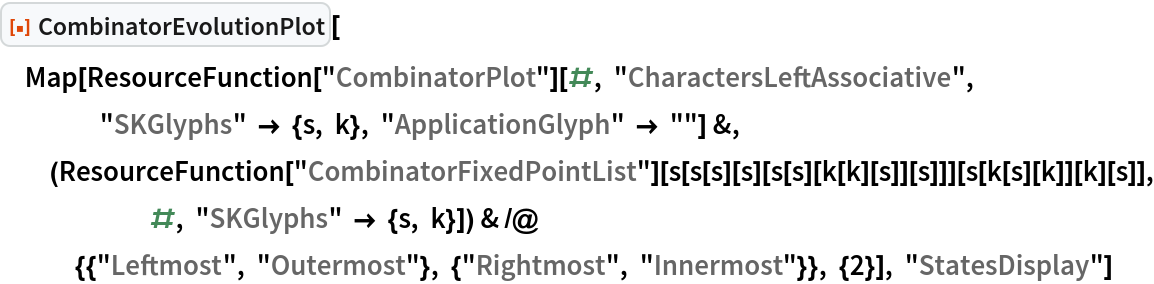Out=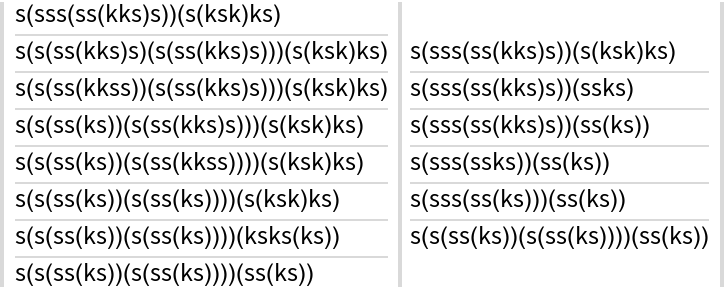Display a combinator evolution with match framing at each step:

 In:=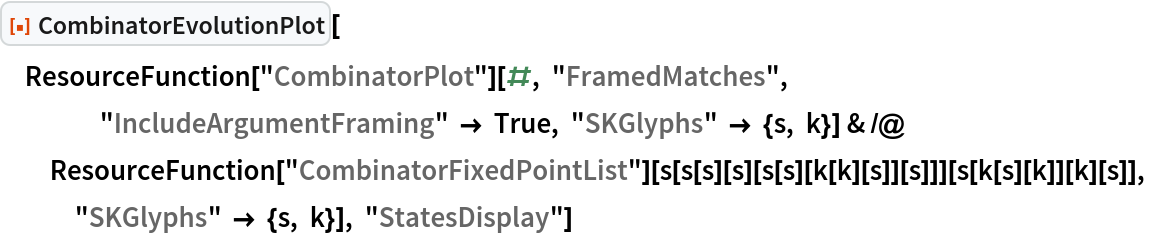Out=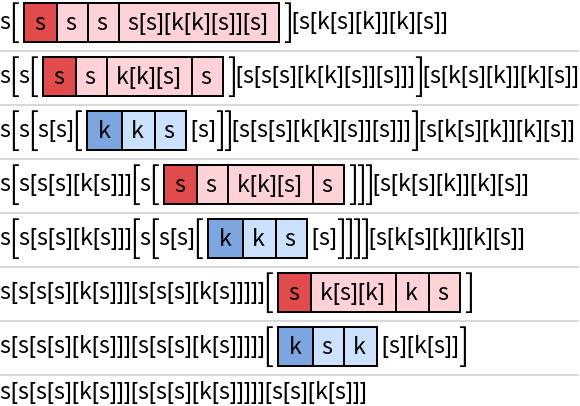Define the color rules for an ArrayPlot of a combinator evolution in reverse Polish notation:

 In:=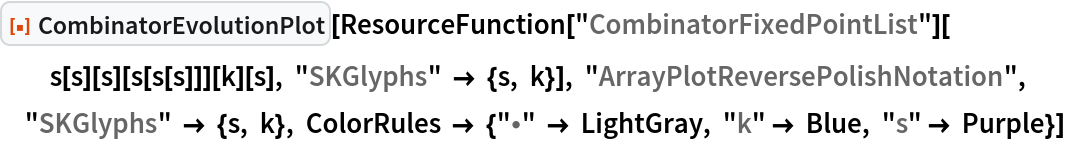Out=